Computer Science - Class 12
Chapter 9 - Structured Query Language

## Points to Note:

• SQL keywords are not case sensitive ie., select and SELECT are the same.
• A semicolon is mandatory at the end of each SQL statement.

## Important SQL Commands:

 COMMAND DESCRIPTION SELECT extracts data from a database UPDATE updates data in a database DELETE deletes data from a database INSERT INTO inserts new data into a database CREATE DATABASE creates a new database ALTER DATABASE modifies a database CREATE TABLE creates a new table ALTER TABLE modifies a table DROP TABLE deletes a table CREATE INDEX creates an index DROP INDEX deletes an index

## QUERIES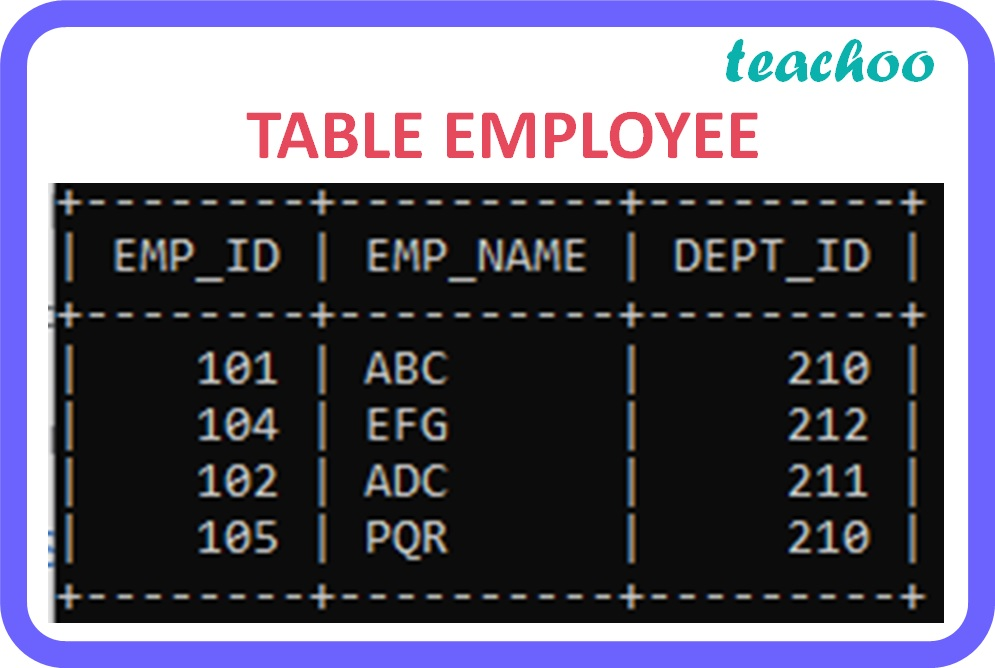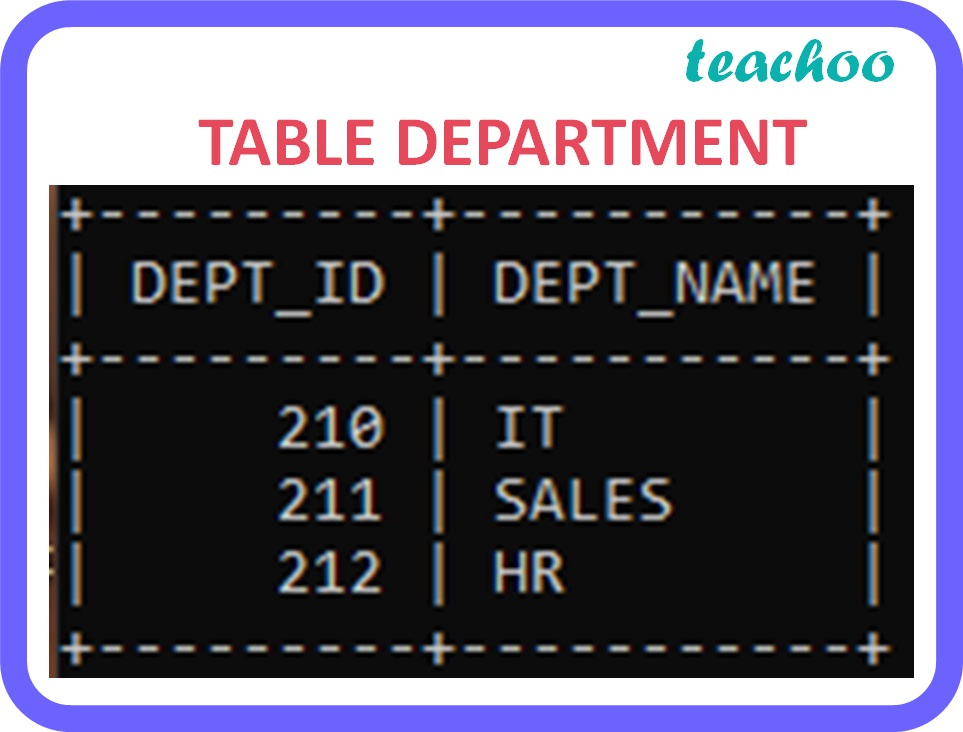QUERY DESCRIPTION OUTPUT ``` SELECT ``` ``` emp_id,emp_name ``` ``` FROM EMPLOYEE ; ``` Displays the mentioned fields of all the records in the table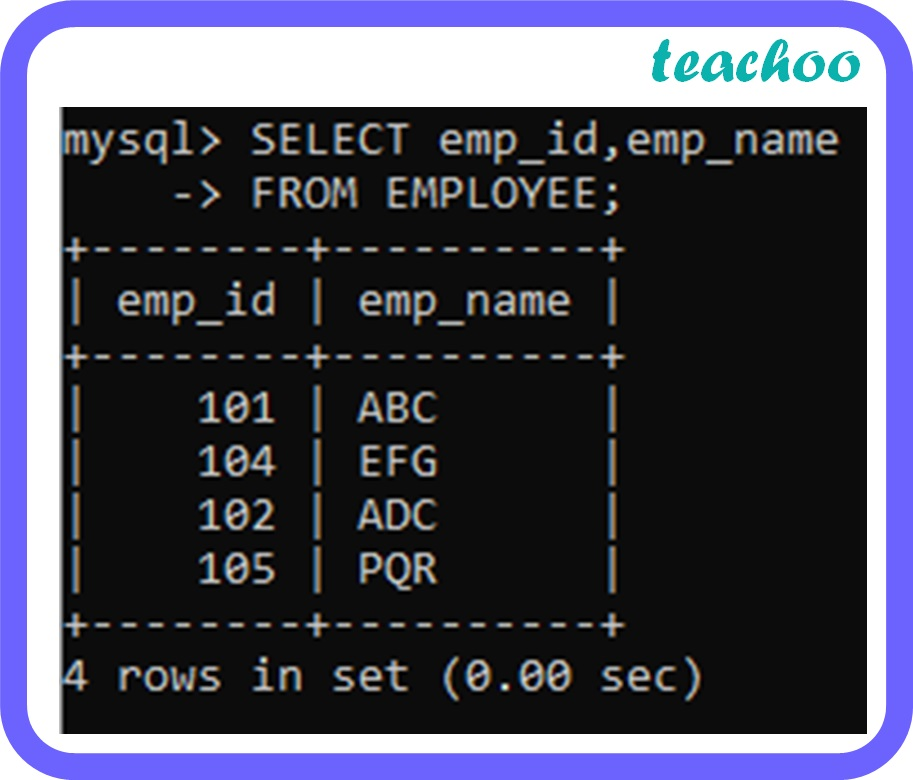``` SELECT * FROM ``` ``` EMPLOYEE ; ``` Displays all the fields of all the records in the table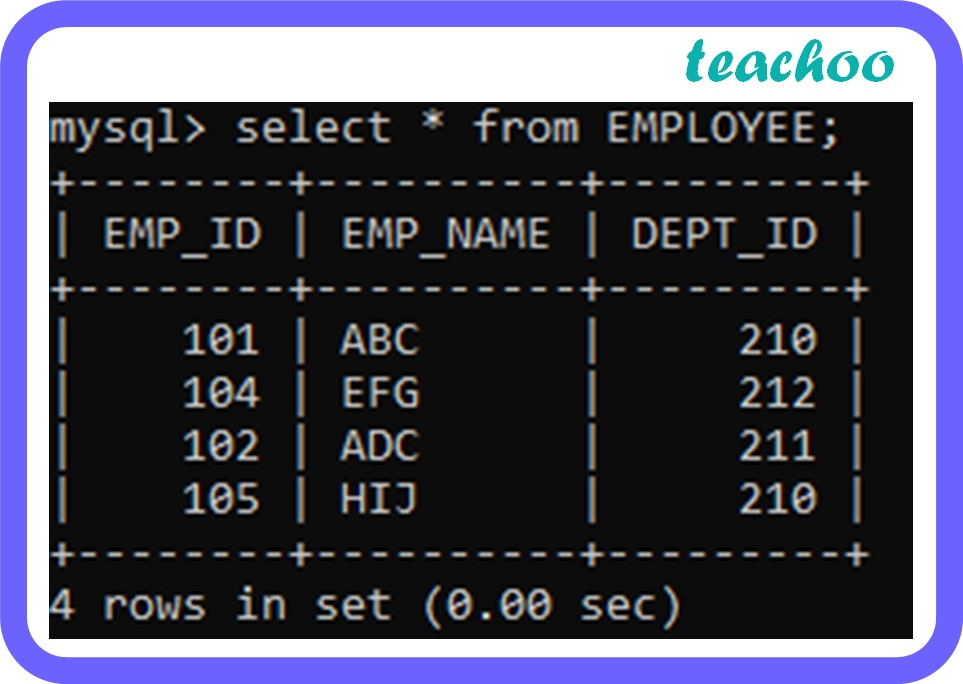``` SELECT DISTINCT ``` ``` dept_id  ``` ``` FROM EMPLOYEE ; ``` Displays the unique values in the mentioned fields of all the records in the table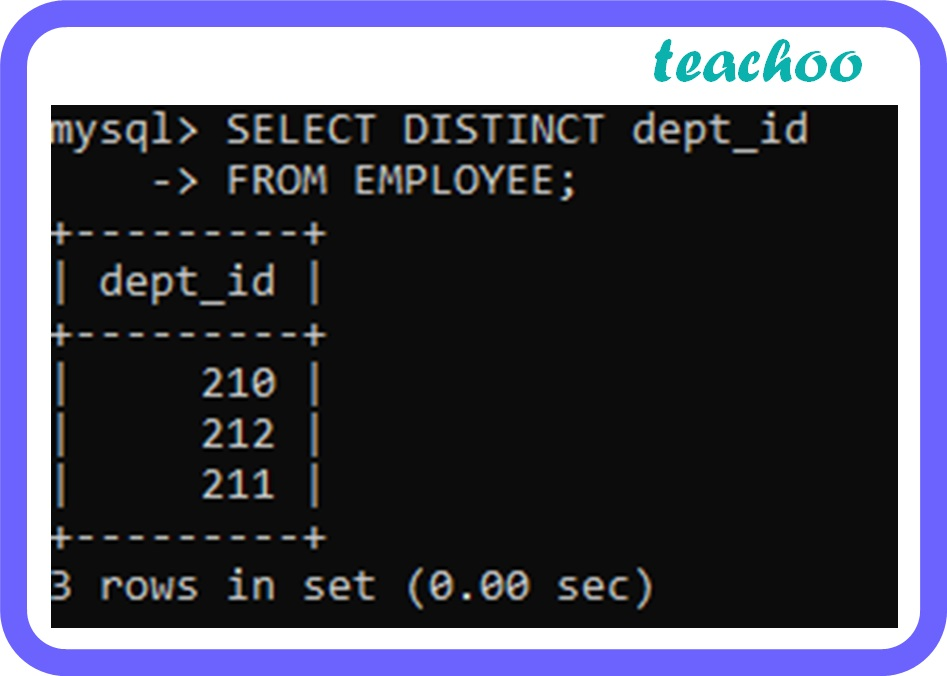``` SELECT emp_name ``` ``` FROM EMPLOYEE ``` ``` WHERE dept_id=210 ; ``` Filters the records based on the condition. Only those records that satisfy the condition mentioned in the WHERE clause is displayed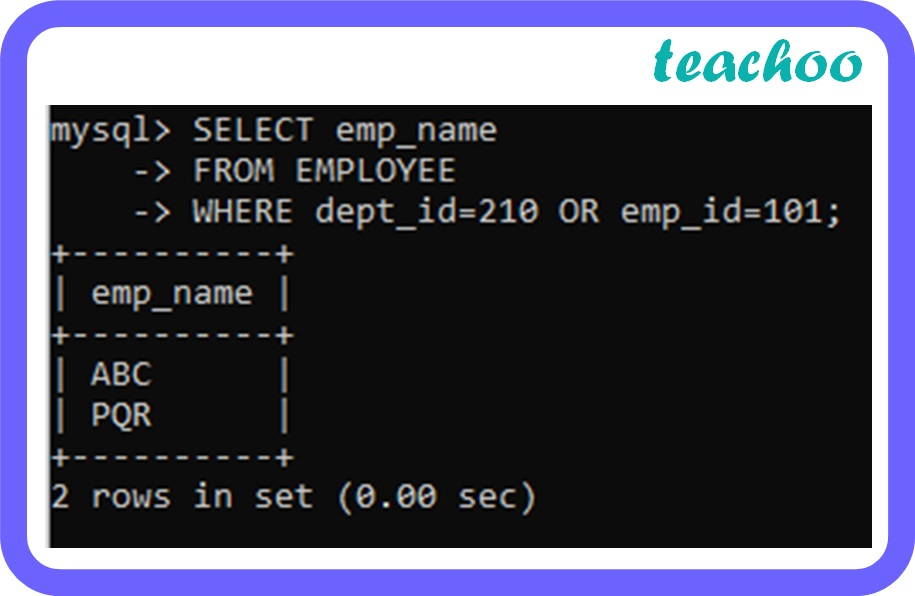``` SELECT emp_name ``` ``` FROM EMPLOYEE  WHERE dept_id=210 AND emp_id=101; ``` Displays all the records in which all the conditions separated by AND are TRUE.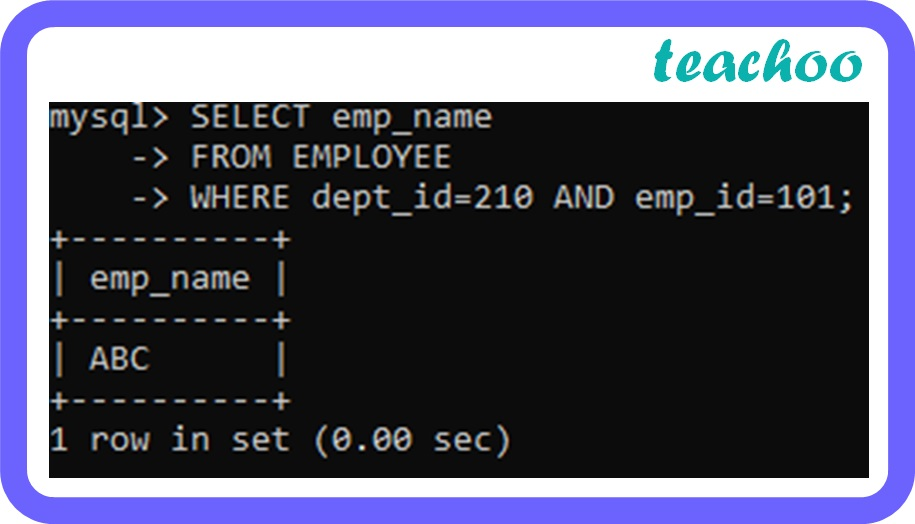``` SELECT emp_name ``` ``` FROM EMPLOYEE ``` ``` WHERE dept_id=210 OR emp_id=101; ``` Displays a record if any of the conditions separated by OR is TRUE.``` SELECT emp_name ``` ``` FROM EMPLOYEE ``` ``` WHERE NOT dept_id=210; ``` Displays a record if the condition(s) is NOT TRUE.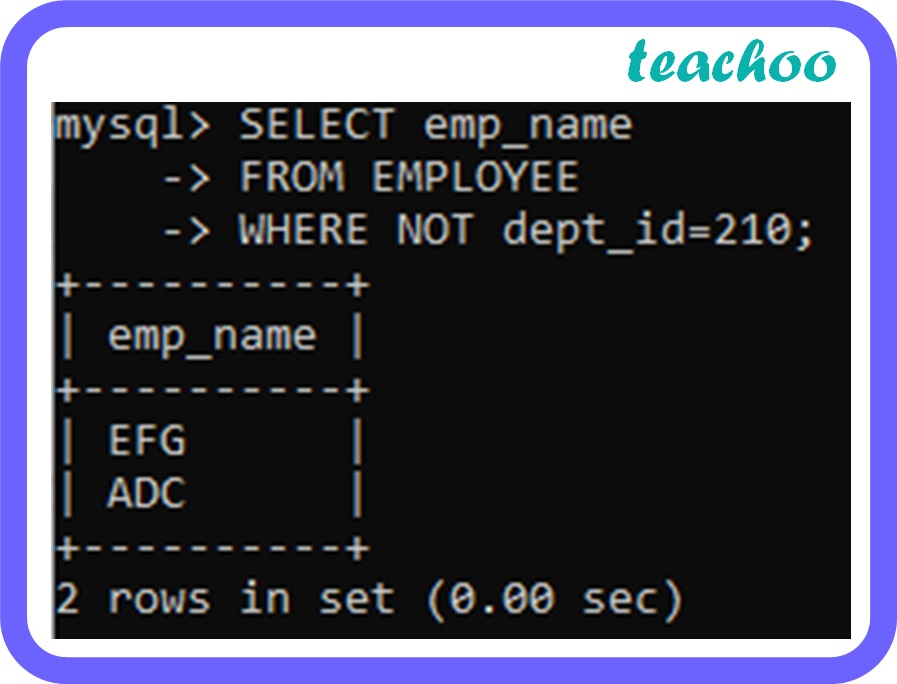``` SELECT emp_id, emp_name ``` ``` FROM EMPLOYEE ``` ``` ORDER BY emp_name ASC ; ``` Displays the records arranged in ascending order of values in the mentioned column.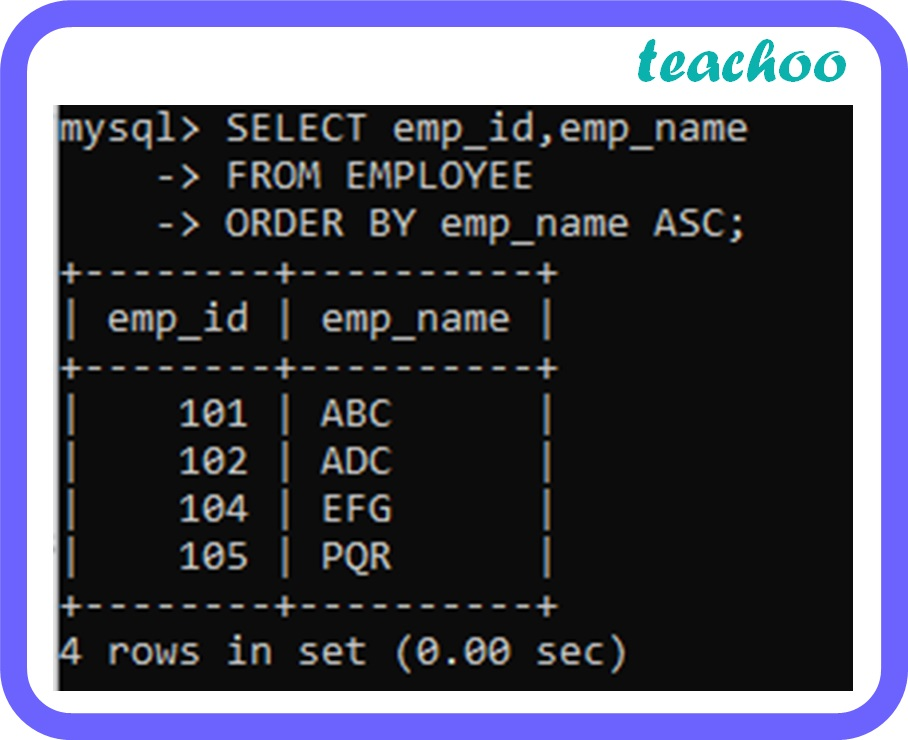``` SELECT emp_id, emp_name FROM EMPLOYEE ``` ``` ORDER BY emp_name DESC ; ``` Displays the records arranged in descending order of values in the mentioned column.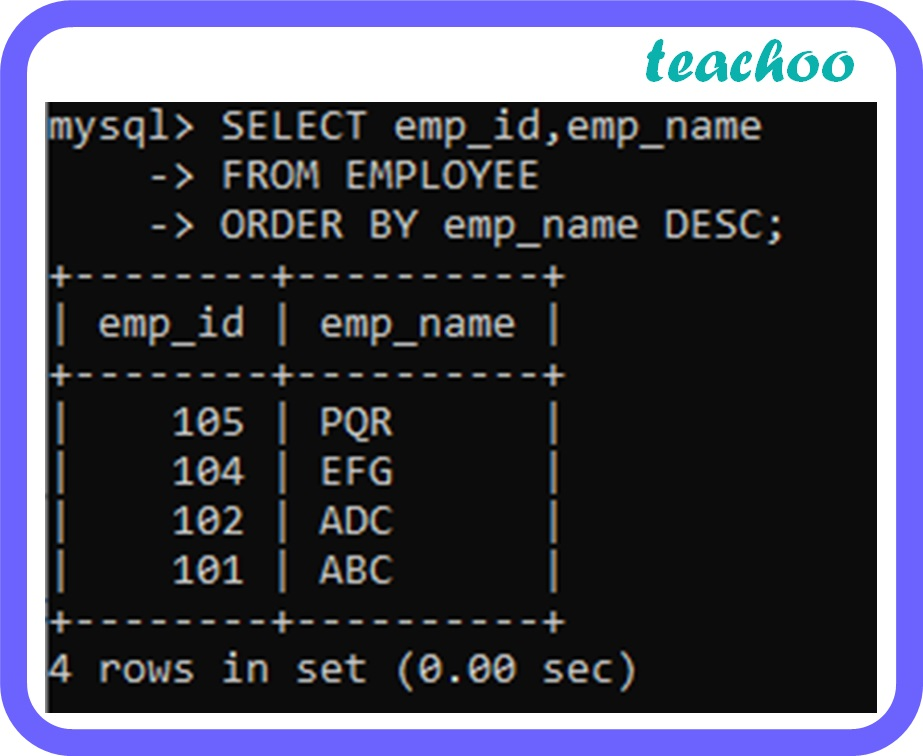``` SELECT emp_name ``` ``` FROM EMPLOYEE ``` ``` WHERE emp_name IS NULL ; ``` Displays the records with NULL value in the mentioned column. ``` SELECT emp_name ``` ``` FROM EMPLOYEE ``` ``` WHERE emp_name IS NOT NULL ; ``` Displays the records with non-NULL value in the mentioned column.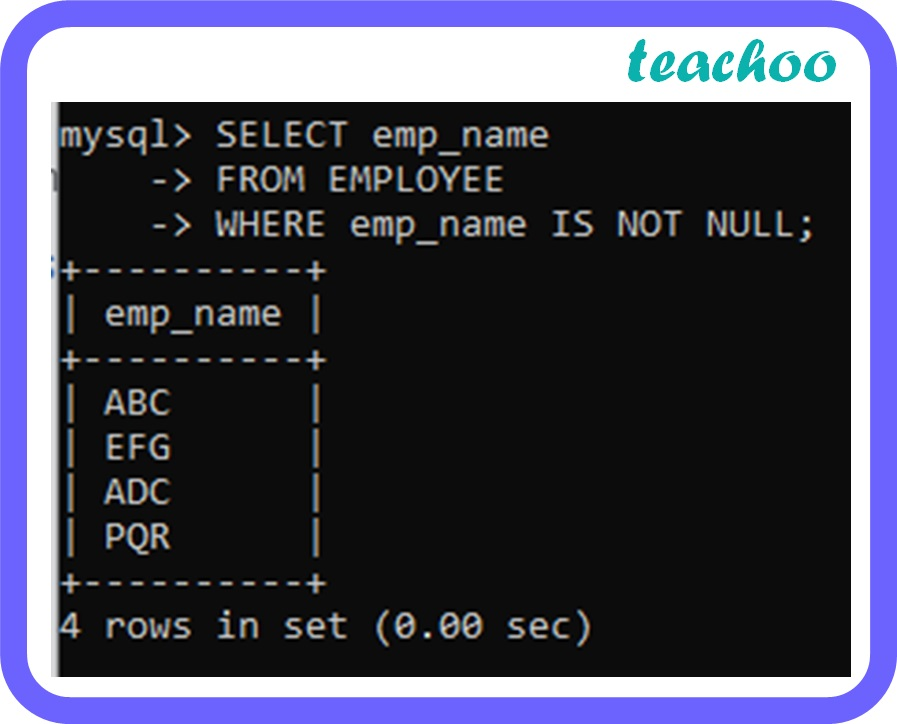``` UPDATE EMPLOYEE ``` ``` SET emp_name = ‘ HIJ’ ``` ``` WHERE emp_id=105; ``` Modifies the existing records in the table.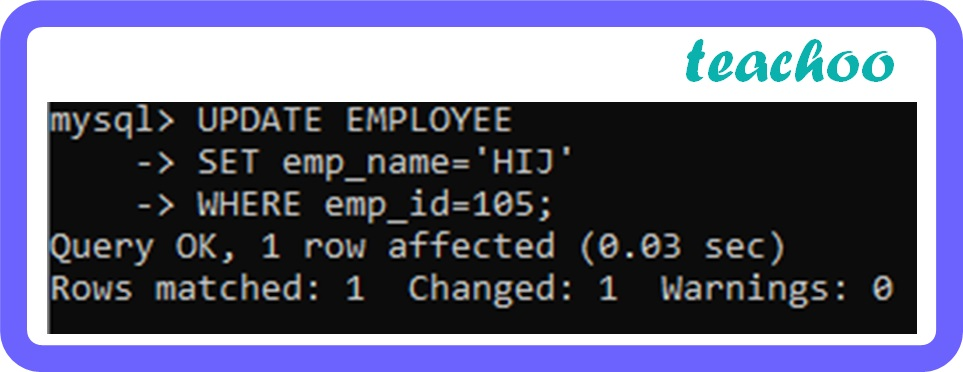Table after executing the statement:``` DELETE FROM EMPLOYEE  ``` ``` WHERE emp_id=105 ; ``` Deletes existing records in the table.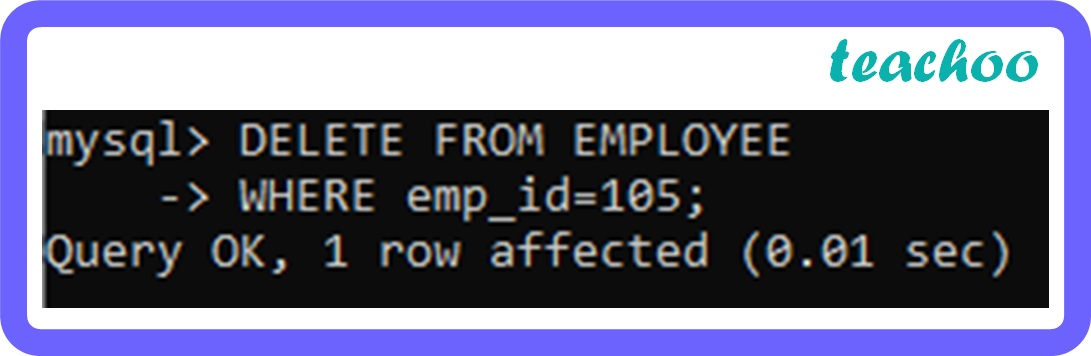Table after executing the statement: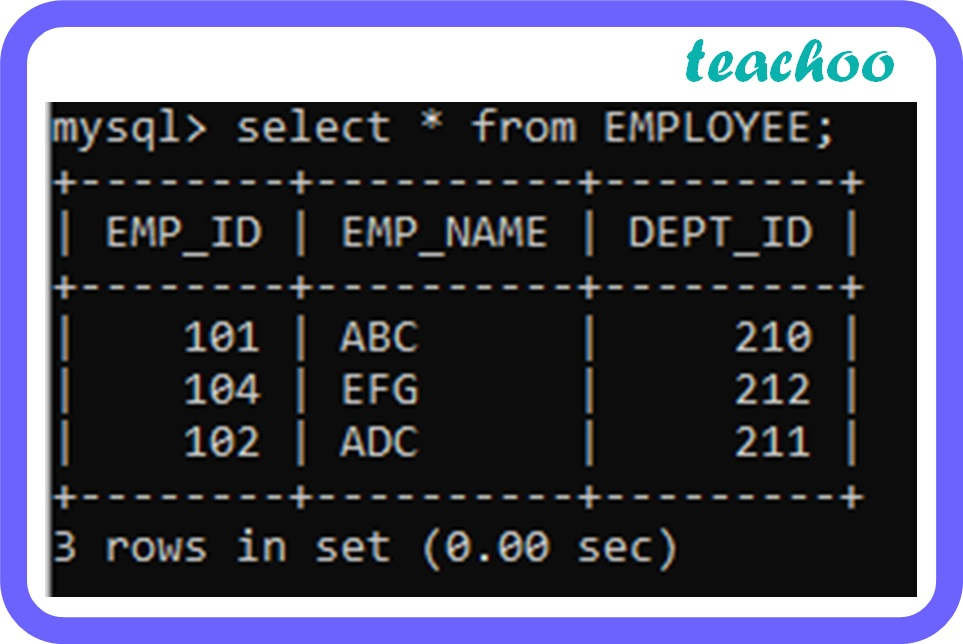``` INSERT INTO EMPLOYEE ``` ``` VALUES ( 105,’PQR’,210 ); ``` Inserts new records into the table.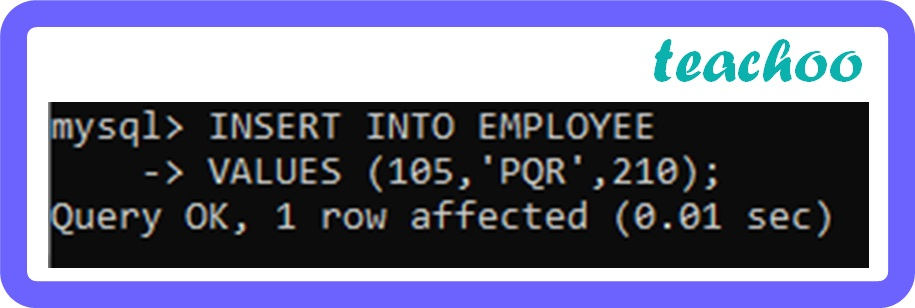Table after executing the statement:``` SELECT MAX( emp_id ) ``` ``` FROM EMPLOYEE ``` ``` WHERE dept_id=210 ; ``` Returns the largest value in the mentioned column.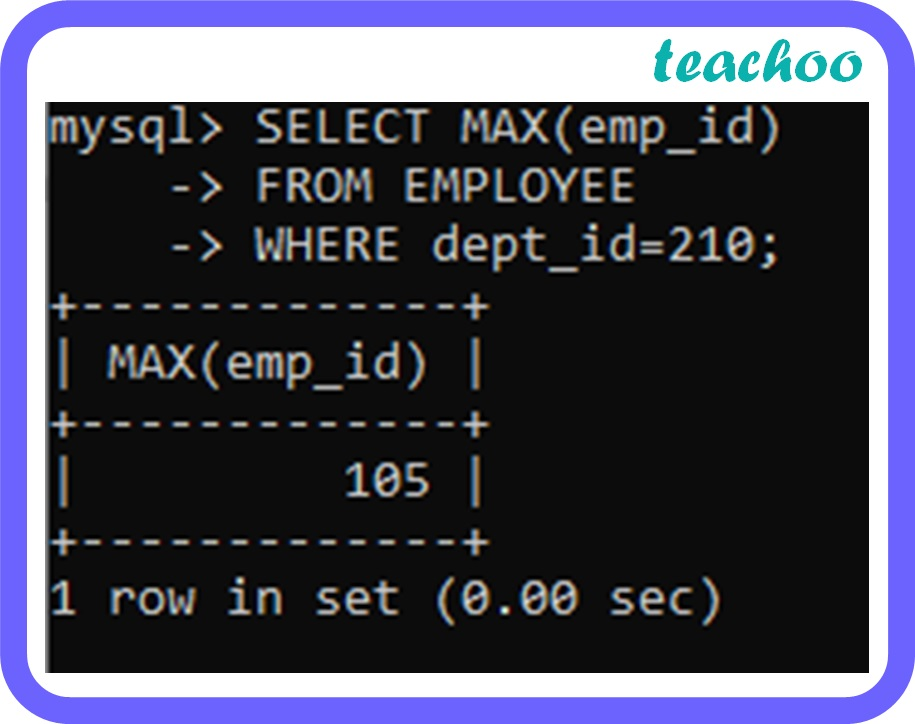``` SELECT MIN( emp_id ) ``` ``` FROM EMPLOYEE ``` ``` WHERE dept_id=210 ; ``` Returns the smallest value in the mentioned column.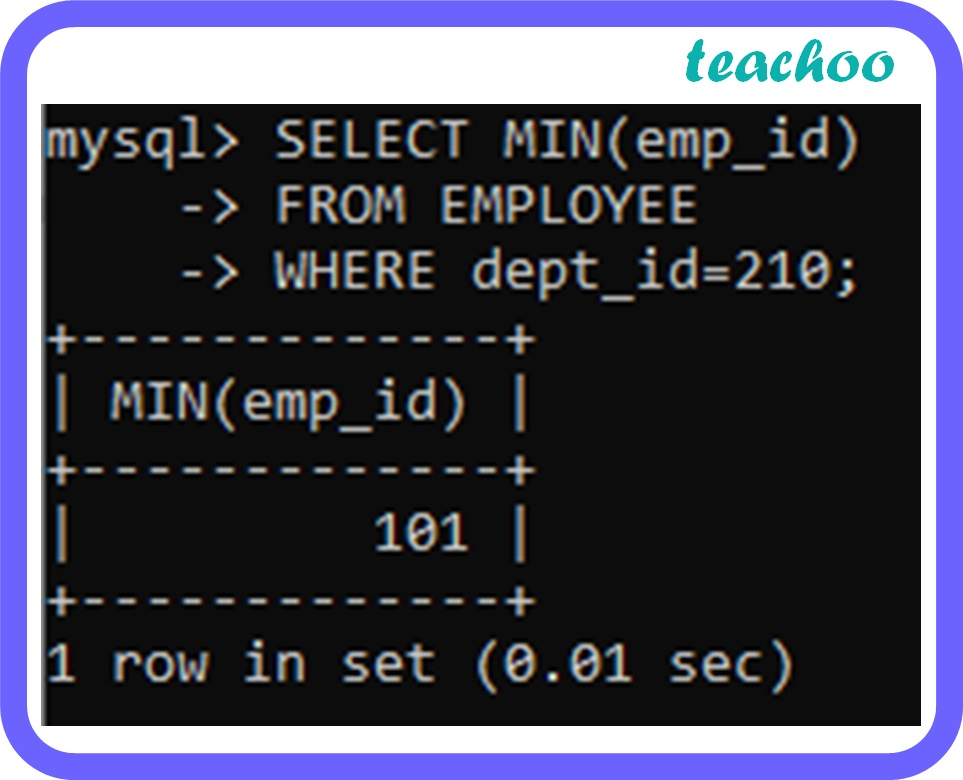``` SELECT COUNT ( * ) ``` ``` FROM EMPLOYEE ``` ``` WHERE dept_id=210 ; ``` Returns the number of rows that match the specified condition.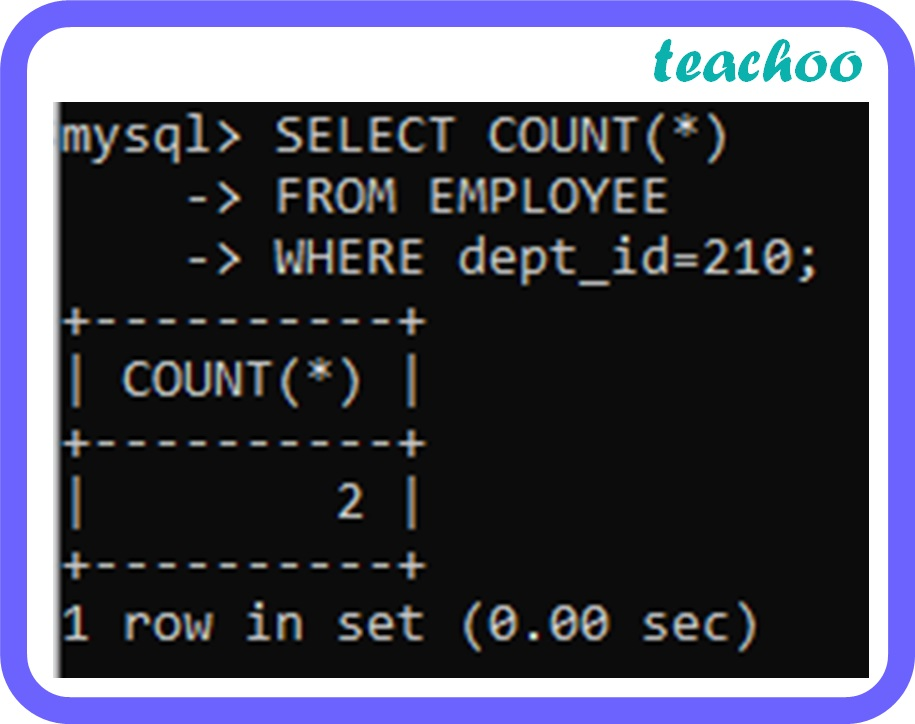``` SELECT column1, column2, ... ``` ``` FROM table_name ``` ``` WHERE columnN LIKE pattern ; ``` Used to search for a specific pattern. The percent sign (%) represents zero, one, or multiple characters The underscore sign (_) represents one, single character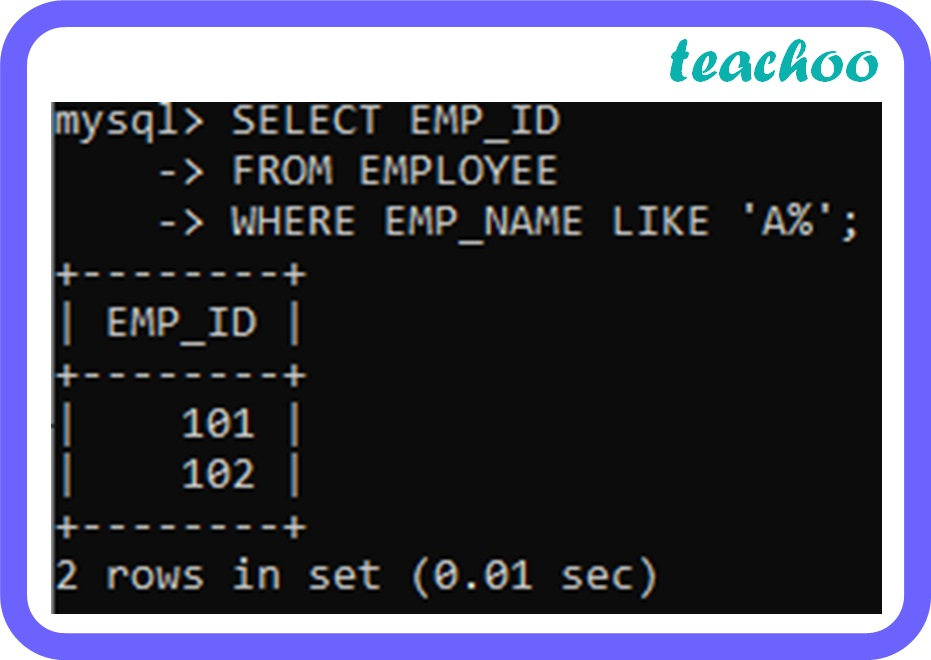``` SELECT column_name(s) ``` ``` FROM table_name ``` ``` WHERE column_name IN ( value1 , value2 , ...); ``` The ‘IN’ operator is used to specify multiple values in the where clause. It is a shorthand for multiple OR conditions.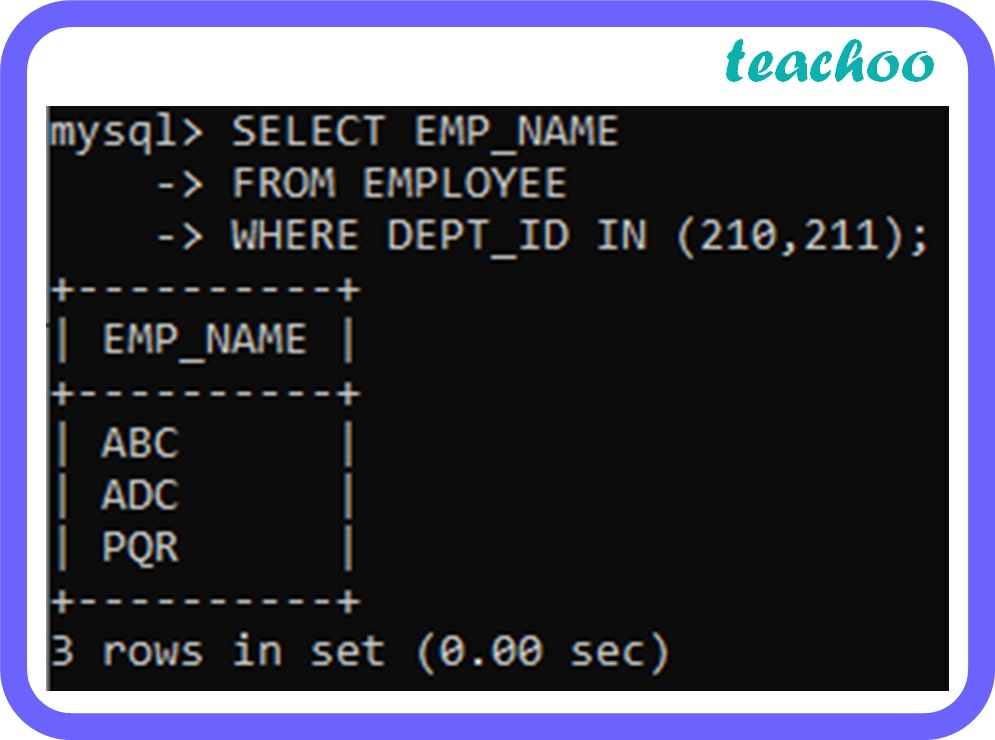``` SELECT column_name(s) ``` ``` FROM table1,table2 ``` ``` WHERE table1.column_name=table2.column_name ; ``` Returns records that have matching values in both the tables.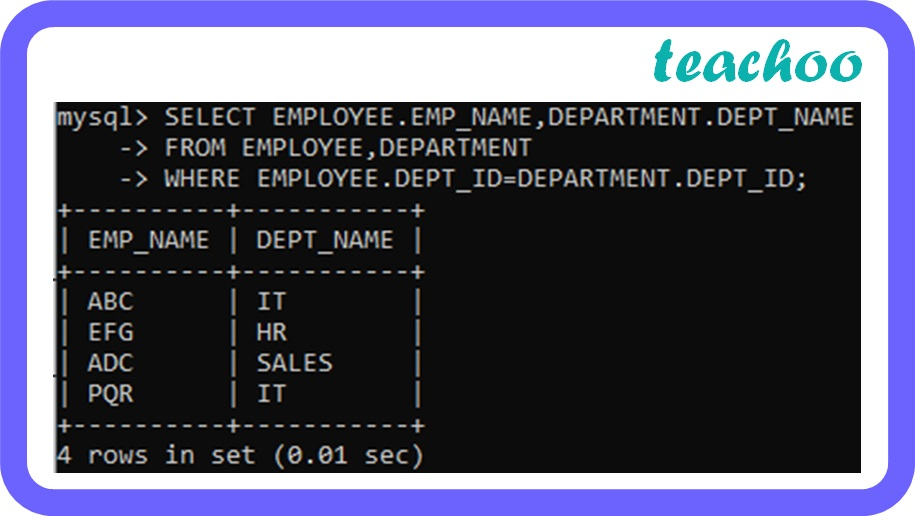``` SELECT column_name(s) ``` ``` FROM table_name ``` ``` WHERE condition ``` ``` GROUP BY column_name(s); ``` Groups rows that have the same values into summary statements. The ‘group by’ statement is often used with aggregate functions like count(), sum(), max(), min(), avg()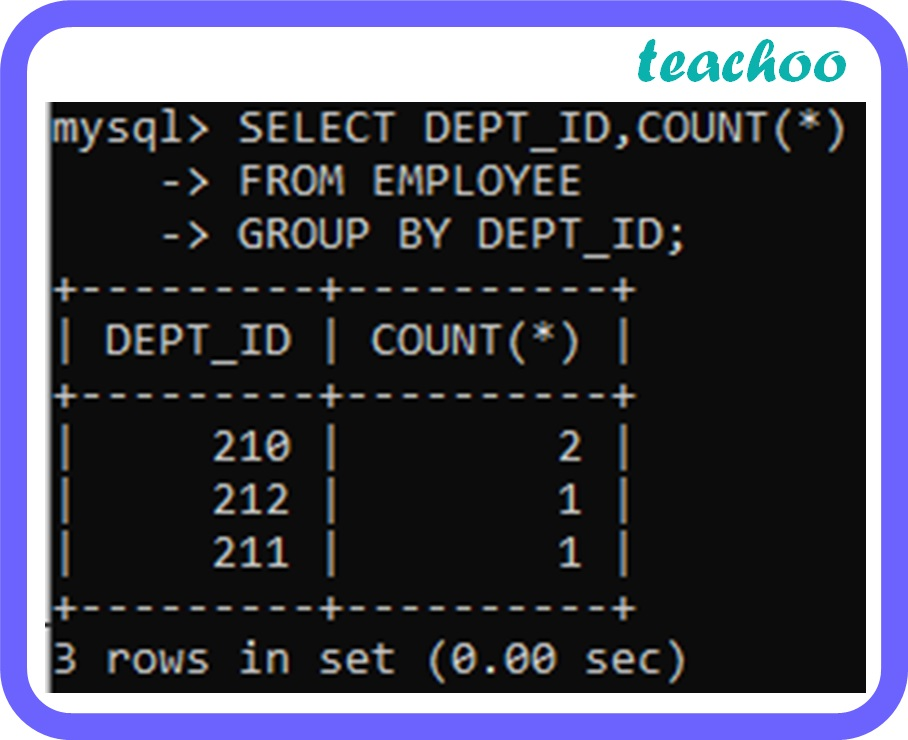``` SELECT column_name(s) ``` ``` FROM table_name ``` ``` WHERE condition ``` ``` GROUP BY column_name(s) ``` ``` HAVING condition; ``` The ‘having clause’ is used to filter the output of the ‘group by’ clause by a specific condition.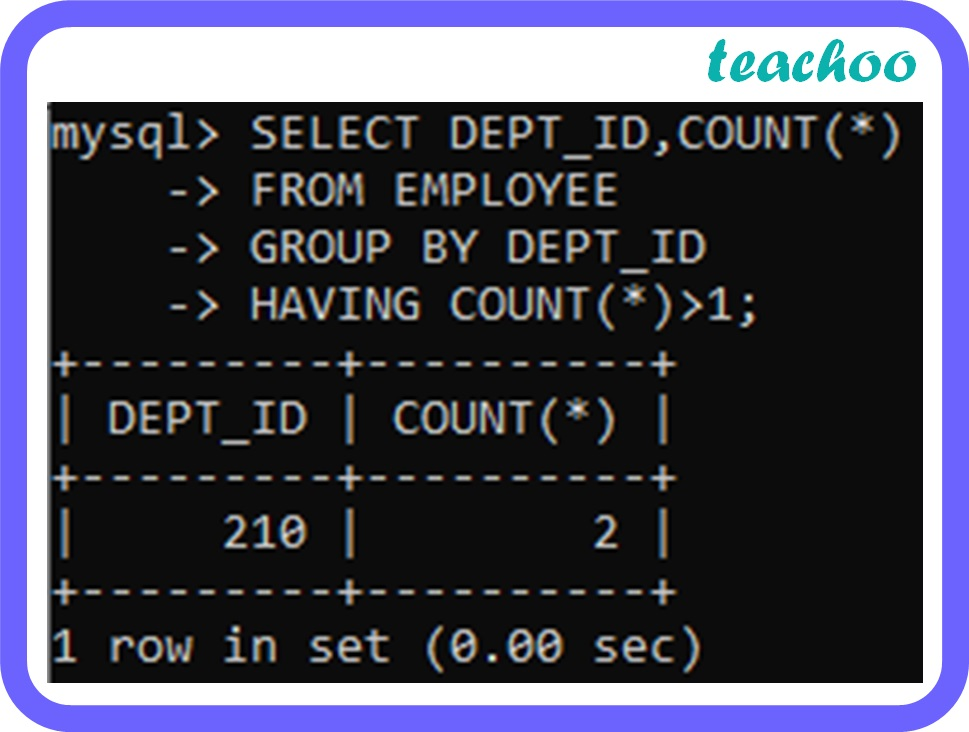Learn in your speed, with individual attention - Teachoo Maths 1-on-1 Class